## Section16.1Static Equilibrium

We say an object is in static equilibrium if none of its parts is accelerating. That means force on each particle of a body in static equilibrium will be zero. Thus, if a body has $N$ particles, then we will have $N$ conditions.

\begin{equation} \vec F_1 = 0,\ \ \vec F_2 = 0, \ \ \vec F_3 = 0,\ \ \cdots,\ \ \vec F_N = 0,\label{eq-static-equilibrium-1}\tag{16.1.1} \end{equation}

where $\vec F_i$ is net force on the $i\text{-th}$ particle.

### Subsection16.1.1Internal and External Forces

Force on each particle in Eq. (16.1.1) is a vector sum of (1) forces from other particles of the body and (2) forces from other objects outside the body. We will call them internal forces and external forces. It will be helpful in our analysis below to separate them.

Suppose we sum up all the internal forces on particle 1 and call it $\vec F_1^{\text{int}}$ and sum up all the external forces on particle 1, call it $\vec F_1^\text{ext}\text{,}$ then we can write the force $\vec F_1$ on particle 1 as

\begin{equation} \vec F_1 = \vec F_1^\text{int} + \vec F_1^\text{ext}.\tag{16.1.2} \end{equation}

Similarly for the forces on the other particles. Using this separation, Eq. (16.1.1) should actually be written as

\begin{align} \vec F_1^\text{int} \amp + \vec F_1^\text{ext} = 0, \label{eq-static-equilibrium-2-1}\tag{16.1.3}\\ \vec F_2^\text{int} \amp + \vec F_2^\text{ext} = 0, \label{eq-static-equilibrium-2-2}\tag{16.1.4}\\ \vec F_3^\text{int} \amp + \vec F_3^\text{ext} = 0, \label{eq-static-equilibrium-2-3}\tag{16.1.5}\\ \amp \vdots \notag\\ \vec F_N^\text{int} \amp + \vec F_N^\text{ext} = 0, \label{eq-static-equilibrium-2-N}\tag{16.1.6} \end{align}

### Subsection16.1.2Static Equilibrium of Rigid Bodies

Every physical body gets deformed, even so slightly, when a force acts on the body. As a consequence of the deformation, a stress develops in the body. We will study these effects in a later section in this chapter. Here, we will work ignore deformations in the body to focus on effect of external forces alone. Objects where this mathematical idealization works well are also called rigid bodies.

Internal forces in Eqs. (16.1.3)$-$(16.1.6) occur as pairs of oppositely directed third law forces. Hence, summing these equations results in their cancellation.

\begin{equation} \vec{F}_1^\text{ext} + \vec{F}_2^\text{ext} + \cdots + \vec{F}_N^\text{ext} = 0.\label{eq-static-equilibrium-net-external-force-sum}\tag{16.1.7} \end{equation}

We can state this as net external force on the rigid body must be zero.

\begin{equation} \vec{F}_\text{net}^\text{ext} = 0.\label{eq-static-equilibrium-net-external-force}\tag{16.1.8} \end{equation}

While Eqs. (16.1.3)$-$(16.1.6) had $N$ vector equations, this equation is only one vector equation. Clearly, balancing of net exernal force does not have all the information in the original set of $N$ vector equation.

Additional relation among the external forces can be obtained by looking at torque of forces on each part of the body. Suppose you pick an arbitrary pivot point P, and calculate the net torque about that point by $\vec \tau = \vec r_P \times \vec F\text{,}$ and sum all the torqus. You will find that the net torque of external forces is zero if the internal forces between two particles act along the line joining them.

\begin{equation} \vec \tau_1^{\text{ext}} + \vec \tau_2^{\text{ext}} + \cdots + \vec \tau_N^{\text{ext}} = 0 \label{eq-static-equilibrium-net-external-torque}\tag{16.1.9} \end{equation}

This gives one more vector equation among external forces. Conditions stated in Eqs. (16.1.8) and (16.1.9) often help us find magnitudes and locations of external forces on a body in equilibrium. They are routinely used to analyze mechanically stable structures as we will see in the worked out examples below.

Simplification for forces in the $xy$ plane only:

Often we find that lines of forces will all be in one plane. We identify this plane with the Cartesian $xy$-plane. The torques of these forces will be either positive $z$ or negative $z$ axis or zero. In these situations, we only have three component equations from the two vector equations, Eqs. (16.1.8) and (16.1.9): $x$ and $y$ components of the net external force and one for the net torque.

\begin{align} \amp F_{1x}^\text{ext}+ F_{2x}^\text{ext} + \cdots + F_{Nx}^\text{ext} = 0, \tag{16.1.10}\\ \amp F_{1y}^\text{ext}+ F_{2y}^\text{ext} + \cdots + F_{Ny}^\text{ext} = 0, \tag{16.1.11}\\ \amp \tau_{1z}^\text{ext} + \tau_{2z}^\text{ext} + \cdots + \tau_{Nz}^\text{ext} = 0. \tag{16.1.12} \end{align}

### Subsection16.1.3Deformable Objects and Static Equilibrium

For an arbitrary structure to be in a static equilibrium, the vanishing of the net external force does not ensure static equilibrium. For instance, if you push on a piece of foam from two opposite sides, the net force on the foam is zero, but the foam gets deformed because of an imbalance between the external and internal forces on the particles at the surface.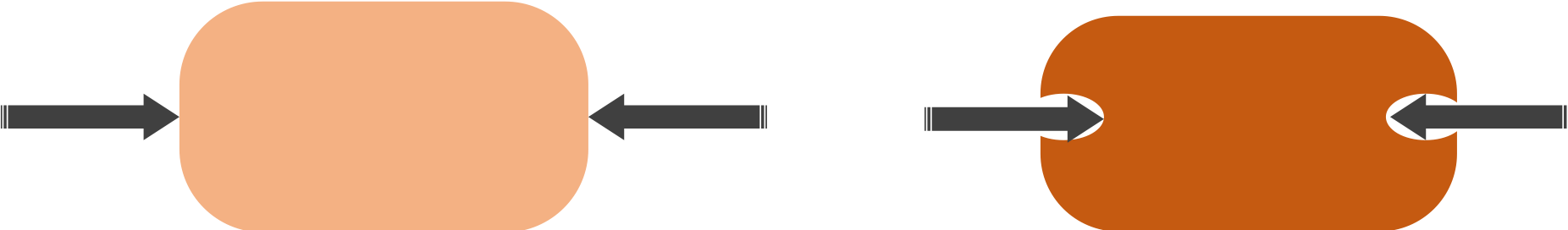Figure 16.1.1. A deformable object will deform under external force. The deformation causes stress to develop in the body. The balancing of internal and external forces occurs at the equilibrium.

While there is no limit on external forces, the internal forces, just like the static frictional force, have an upper limit and can be overcome by a large enough external force. Therefore, to study the equilibrium of a deformable object, we need the complete condition for the static equilibrium given in Eq. (16.1.3)$-$(16.1.6), viz., the net force on each and every particle of the system must vanish independently, not just a vector sum of them.

To be in static equilibrium, the external forces must balance the internal forces at each point. In this way, the analysis of the static equilibrium of deformable systems provides us with an understanding of the strength of the material. In this chapter, we will study equilibrium with regard to the external forces as well as internal forces.

But, for simplicity, we will look at the problems for the ideal undeformable objects first. This would be a review of forces and torques you have studied in earlier chapters.

A $10\text{-kg}$ wooden plank is placed on two bathroom scales separated by a distance $3.0\text{ m}$ as shown in Figure 16.1.3. A man of mass $100\text{ kg}$ stands on the plank $1.0\text{ m}$ from one of the scales, say the scale on the left.

What are the readings on the two scales?

Hint

Balance the net torque on the plank about each end. Scale reading are given by force divided by $g\text{.}$

$71.7\text{ kg}$ and $38.3\text{ kg}\text{.}$

Solution

There are four systems here - the two scales, the plank, and you. The only system that everything interacts with is the plank. So, we will try to balance forces and torques on this.

The forces on the plank are shown in Figure 16.1.4. The normal forces from the scales are the readings in those scales. so, we need to find them. We can set up balance of forces and torques. However, we can also set up just the balance of torques about two different pivot points giving us the two independent equations for the two unknowns.

Method 1: Set up two torques equations.

Let's first work out the weight values since we will need them in our calculations below.

\begin{gather*} W_1 = m g = 10\text{ kg}\times 9.81\text{ m/s}^2 = 98.1\text{ N}.\\ W_2 = M g = 100\text{ kg}\times 9.81\text{ m/s}^2 = 981\text{ N}. \end{gather*}

From balancing torques about the left end we get

\begin{equation} -W_1 \times 1.5\text{ m} - W_2\times 1.0\text{ m} + F_R\times 3\text{ m} = 0,\label{eq-stat-eq-weights-torque-1}\tag{16.1.13} \end{equation}

and about the right end we get

\begin{equation} W_1 \times 1.5\text{ m} + W_2 \times 2.0\text{ m} - F_L\times 3\text{ m} = 0,\label{eq-stat-eq-weights-torque-2}\tag{16.1.14} \end{equation}

where I have used the convention that counterclockwise sense is positive and clockwise sense negative. From these two equations, we get the readings we get in the two scales.

\begin{align*} F_L \amp = 703.0\text{ N}, \\ F_R \amp = 376.0\text{ N}. \end{align*}

The scale readings show these numbers divided by $g=9.81\text{ m/s}^2\text{.}$ Therefore scale readings are

\begin{align*} S_L \amp = F_L/g = 71.7\text{ kg}, \\ S_R \amp= F_R/g = 38.3\text{ kg}. \end{align*}

Method 2: Set up one torque equation and one force balance equation.

The force balance equation gives

\begin{equation} F_L + F_R = 10\text{ kg}\times 9.81\text{ m/s}^2 + 100\text{ kg}\times 9.81\text{ m/s}^2 = 1,078\text{ N},\label{eq-stat-eq-weights-force-1}\tag{16.1.15} \end{equation}

and the torque balance equation about, say the left end gives Eq. (16.1.13). From the torque balance we got

\begin{equation*} F_R = 376.0\text{ N}. \end{equation*}

which we use in the force balance equation, Eq. Eq. (16.1.15). This gives

\begin{align*} F_L \amp = 1,079\text{ N} - F_R \\ \amp = 703\text{ N}. \end{align*}

These will give the same values for the readings as by method 1.

A man holds a $90\text{-kg}$ weight, about 20 pounds, such that his forearm makes a $60^{\circ}$ angle with the upper arm and $40^{\circ}$ angle with the horizontal dorection. Assuming that the tension in the biceps provide the necessary force that balances the torque about the pivot point shown as a dot at the corner of elbow. What will be the tension $F_B$ in the biceps?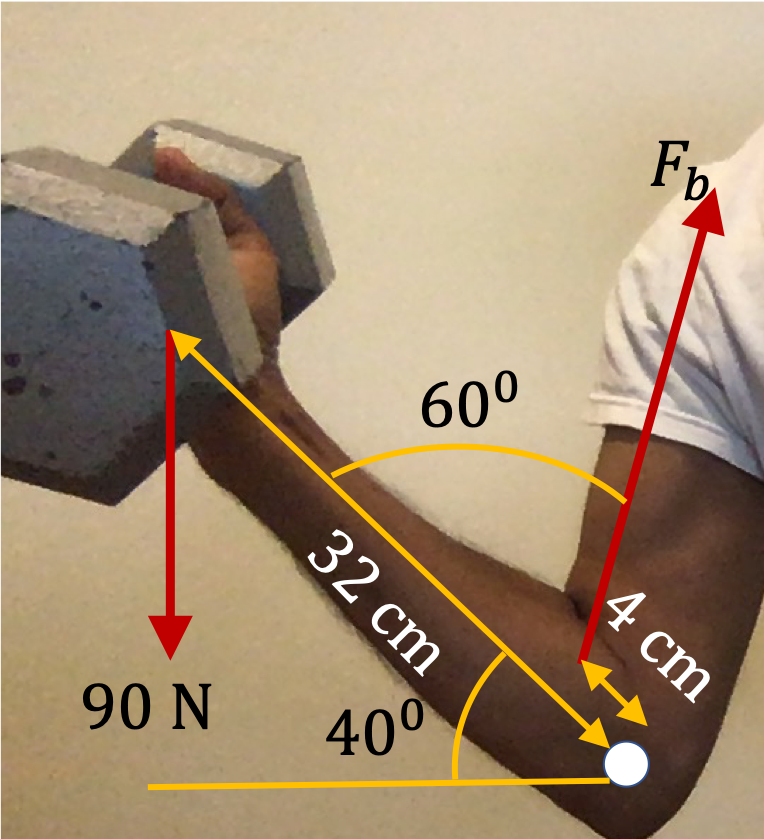Hint

Balance the torques.

$637.3\text{ N}\text{.}$

Solution

From the given picture, we can deduce Figure 16.1.6 for computing the lever arms of the two forces involved.

The lever arms are

\begin{align*} r_{90} \amp = 32\times \cos(40^\circ) = 3.46\text{ cm},\\ r_{fb} \amp = 4\times \sin(60^\circ) = 24.5\text{ cm}. \end{align*}

Therefore, balancing the torques of the two forces about the pivot O gives

\begin{equation*} 3.46\, F_{B} = 24.5\times 90. \end{equation*}

This gives

\begin{equation*} F_{B} = 637.3\text{ N}. \end{equation*}

A ladder of length $L$ and of mass $M$ slides when the ladder leans against a frictionless wall at an angle greater than $\theta$ from the floor.

(a) What is the magnitude of the static frictional force between the ladder and the floor?

(b) What is the magnitude of the normal force from the floor?

(c) What is the magnitude of the normal force from the wall?

(d) What is the coefficient of static frictional force between the ladder and the floor?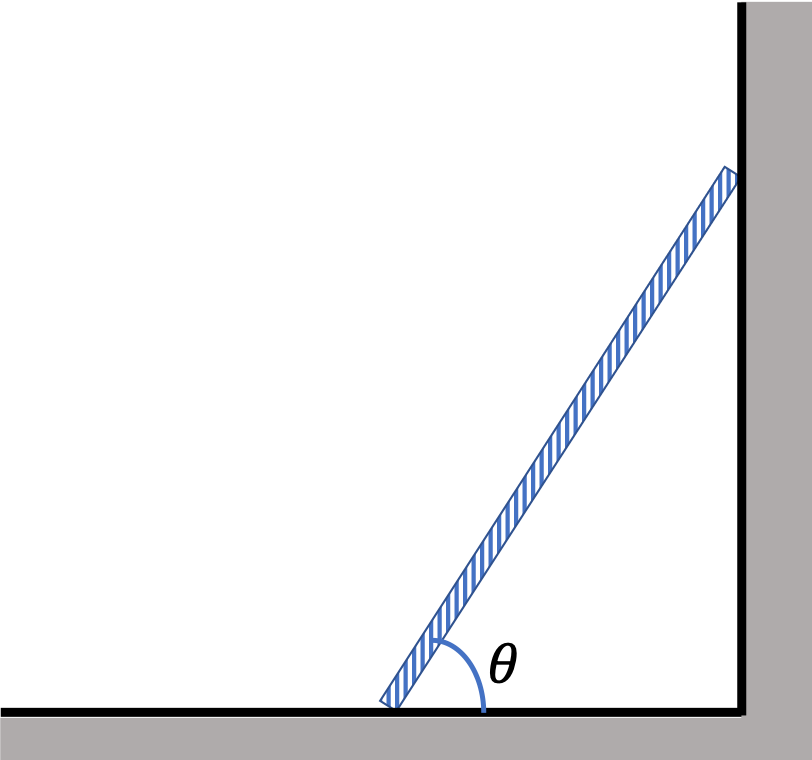Hint

Start with identifying forces, then use free body diagram to set up zero net force, and then pick a pivot point and implenent the zero torque equation.

(a) $S_f = \frac{W}{2} \cot\theta\text{,}$ (b) $N_f = W \text{,}$ (c) $N_w = \frac{W}{2} \cot\theta\text{,}$ (d) $\frac{1}{2} \cot\theta \text{.}$

Solution

Let us first draw a picture of the situation and locate all the forces on the ladder. Then we will extract a free-body diagram from this diagram for writing out the equations corresponding to the balance of the external forces. To calculate the torque of the forces, the free-body diagram is not useful. For the torque calculation, we will go back to the original force diagram and choose a convenient pivot point. The choice of pivot point is arbitrary since the net force is zero.

The diagram of forces on the ladder shows various external forces on the ladder and the place those forces act on the ladder. This is given in Figure 16.1.8. Note that there are two normal forces on the ladder, one from the floor and the other from the wall. Similarly, there are two frictional forces on the ladder, one from the floor and the other from the wall. The problem states that the frictional force from the wall is zero.

One more thing to note here is that I had make a decision about the direction of the static frictional force. Since, the ladder is not accelerating with respect to the ground, I anticipated that this frictional force must balance the normal force from the wall, and that is why I choose the frictional force to be in that direction.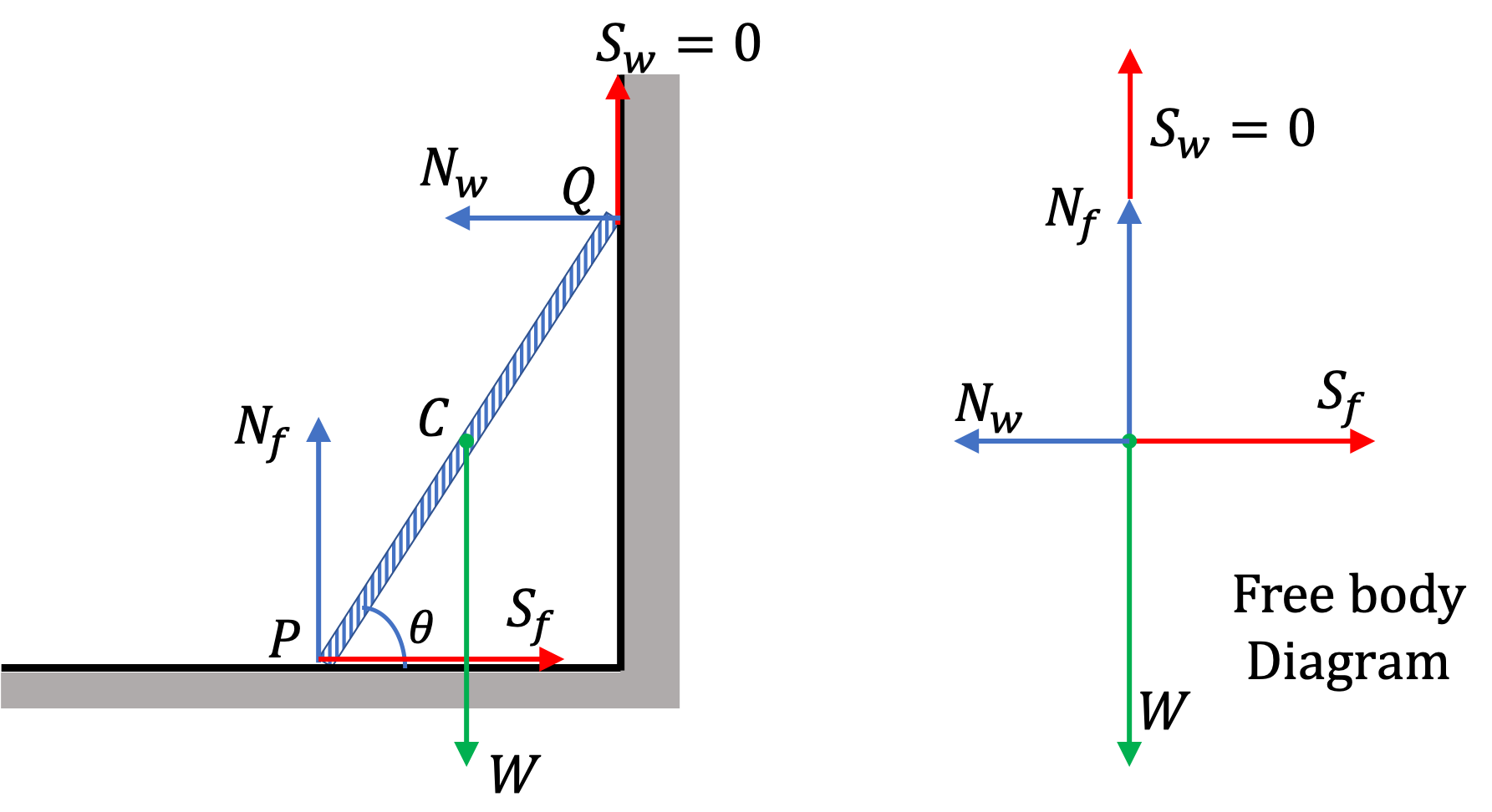Figure 16.1.8. Left panel shows various forces on the ladder. The right panel shows the free-body diagram. Since, the forces are oriented only in perpendicular directions, we take one direction to be the $x$ axis and the other $y$ axis. Here $W$ is the weight, $N$ the normal force and $S$ the static friction force.

The zero net force equation has two components, which is quite easy to work out here. We get the following relations among the magnitudes.

\begin{gather} S_f-N_w = 0,\label{eq-safety-in-ladder-static-eq-normal-wall}\tag{16.1.16}\\ N_f-W = 0.\tag{16.1.17} \end{gather}

Therefore,

Since the net force is zero, the choice of pivot point for torque equation would be arbitrary. In the static problems, we choose a pivot point so that the lever arm for some of the unknown forces would be zero for that choice. In the present case, we notice that if we choose the pivot point at the point where the ladder touches the ground, i.e., point P in Figure 16.1.8, and then the torques from two of the forces, viz, $N_f$ and $S_f$ will be zero. Let $l_{nw}$ and $l_w$ be the lever arms of forces $N_w$ and $W$ respectively, about pivot point P. Then, the torque equation gives

\begin{equation} N_w\ L_{nw} - W \ L_w = 0.\label{eq-safety-in-ladder-torque-zero}\tag{16.1.19} \end{equation}

The lever arms can be written in terms of the length $L$ of the ladder and the angle $\theta$ the ladder makes with the horizontal.

\begin{align*} \amp L_{w} = \frac{L}{2}\cos\theta, \\ \amp L_{nw} = L\sin\theta. \end{align*}

Substituting in the torque equation, Eq. (16.1.19), we find the following.

\begin{equation} N_w\ \sin\theta - \frac{W}{2} \cos\theta = 0.\tag{16.1.20} \end{equation}

Therefore,

\begin{equation} N_w = \frac{W}{2} \cot\theta.\tag{16.1.21} \end{equation}

This in Eq. (16.1.16) gives

\begin{equation} S_f = \frac{W}{2} \cot\theta.\tag{16.1.22} \end{equation}

Finally, since the condition of the ladder is such that the static friction force on the ladder is the maximum, we have

\begin{equation*} S_f^{\text{max}} = \frac{W}{2} \cot\theta. \end{equation*}

From this and the normal force from floor we get the static friction coeffiecient at the floor.

\begin{equation} \mu_{f,s} = \dfrac{S_f^{\text{max}}}{N_f} = \frac{1}{2} \cot\theta.\tag{16.1.23} \end{equation}

Now we summarize the findings. (a) $S_f = \frac{W}{2} \cot\theta\text{,}$ (b) $N_f = W \text{,}$ (c) $N_w = \frac{W}{2} \cot\theta\text{,}$ (d) $\frac{1}{2} \cot\theta \text{.}$

A beam that extends beyond its support is called a cantilever. Consider a cantilever of length $L$ and mass $M$ resting and bolted to two supports, one at the end and the other is at a distance $D$ from it as shown in Figure 16.1.10.

(a) Find the conditions on the forces such that the cantilever is in static equilibrium.

(b) For $L = 30\text{ m}\text{,}$ $M = 1000\text{ kg}\text{,}$ and $D = 12\text{ m}\text{,}$ find the magnitude and directions of the forces on the beam from the supports.

Hint

(a) Balance forces and torque, (b) Use numbers in the results (a).

(a) Horizontal and vertical forces from the left post : $F_{11} = 0\text{,}$ $F_{12} = Mg\left(1-\frac{L}{2D} \right)\text{;}$ Vertical force from the second post $F_2 = \left(\frac{L}{2D} \right)Mg\text{.}$ (b) $0,\ -2500\text{ N},\ 12000\text{ N}\text{.}$

Solution

(a) The beam has the forces from the support and the bolt at one end, an upward normal force from the support in the middle, and the force of gravity from the Earth as shown in Figure 16.1.11.

To simplify, we will combine the forces of the bolt and the support at the end into a horizontal force $\vec F_{11}$ and a vertical force $\vec F_{12}\text{,}$ the sum of the two forces at the end give us the net force $\vec F_1$ at that point.

\begin{equation*} \vec F_1 = \vec F_{11} + \vec F_{12}. \end{equation*}

Normally, one writes the force $\vec F_{11}$ as $\vec F_{1x}$ and $\vec F_{12}$ as $\vec F_{1y}\text{.}$ This notation is confusing since symbols $F_{1x}$ and $F_{1y}$ stand for $x$ and $y$ -=components of the vector $\vec F_1$ and are not vectors themselves. Therefore, we will use $\vec F_{11}$ and $\vec F_{12}$ for the the horizontally and vertically pointed vectors whose sum is the force at the end.

Based on physical grounds we expect that all four forces $\vec F_{11}\text{,}$ $\vec F_{12}\text{,}$ $\vec F_2$ and the weight $\vec W$ will be in one plane, which we take to be the $xy$-plane of the coordinate system shown. Balancing the forces immediately gives the following equations.

\begin{align} \amp F_{11} = 0, \label{eq-cantilever-1}\tag{16.1.24}\\ \amp F_{12}+F_2 - W = 0. \label{eq-cantilever-2}\tag{16.1.25} \end{align}

Balancing the torques about O gives the following equation.

\begin{equation} D F_2 = \frac{1}{2}LMg.\label{eq-cantilever-3}\tag{16.1.26} \end{equation}

Equations (16.1.24)$-$(16.1.26) can be solved to yield the following for the magnitudes of the forces.

\begin{align} \amp F_{11} = 0,\tag{16.1.27}\\ \amp F_{12} = Mg\left(1-\frac{L}{2D} \right),\label{eq-cantilever-force-bolt}\tag{16.1.28}\\ \amp F_2 = \left(\frac{L}{2D} \right)Mg. \tag{16.1.29} \end{align}

$F_{11} = 0$ says that there is no horizontal component of force $\vec F_1$ at the end, and Eq. (16.1.28) says that if the second support is less that $L/2$ from the end, then the force in the vertical direction at the end support is downward, i.e. the force there is dominated by the push back from the bolt at the top of the beam.

(b) Plugging in the numerical values we find the following.

\begin{align*} \amp F_{11} = 0, \\ \amp F_{12} = -2,500\text{ N},\\ \amp F_2 = 12,000\text{ N}, \end{align*}

where I have rounded off the magnitudes to the two significant digits.

A sign of mass $300\text{ kg}$ is hanging $1.0\text{ m}$ from the end of a horizontal beam of mass $50\text{ kg}$ and length $3\text{ m}$ held in place as shown in the figure. The cable makes an angle of $30^\circ$ with the beam.

(a) Find the magnitudes of the tension in the cable and the force applied by the pin.

(b) What is the direction of the force by the pin?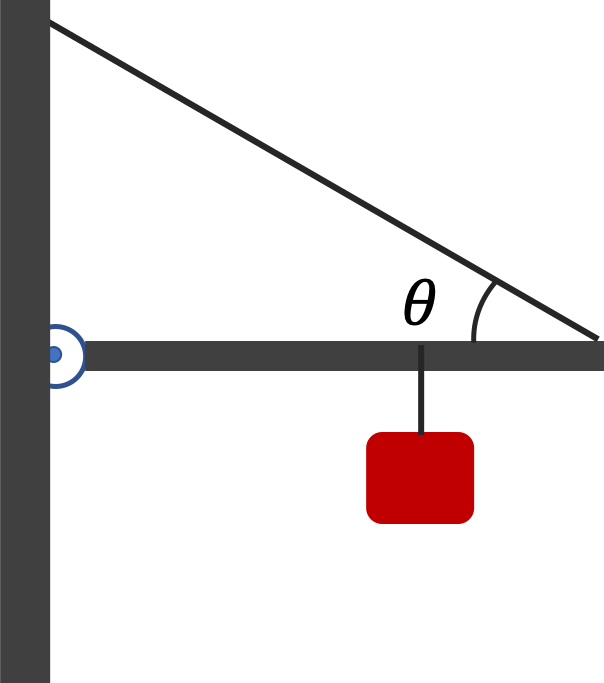Hint

First draw a diagram with all forces on the beam. Then apply force and torque balancing.

(a) $T=4,415\text{ N}\text{,}$ $F = 4,015\text{ N}\text{,}$ (b) $17.8^\circ\text{.}$

Solution 1 (a)

The forces on the beam are shown in the figure. We use $x$ axis horizontal and $y$ axis along vertical. We denote the components of the force by the pin by $F_x$ and $F_y\text{,}$ from which we would get the magnitude $F = \sqrt{ F_x^2 + F_y^2 }\text{.}$ We will implement the balancing of force components and torque about the pin.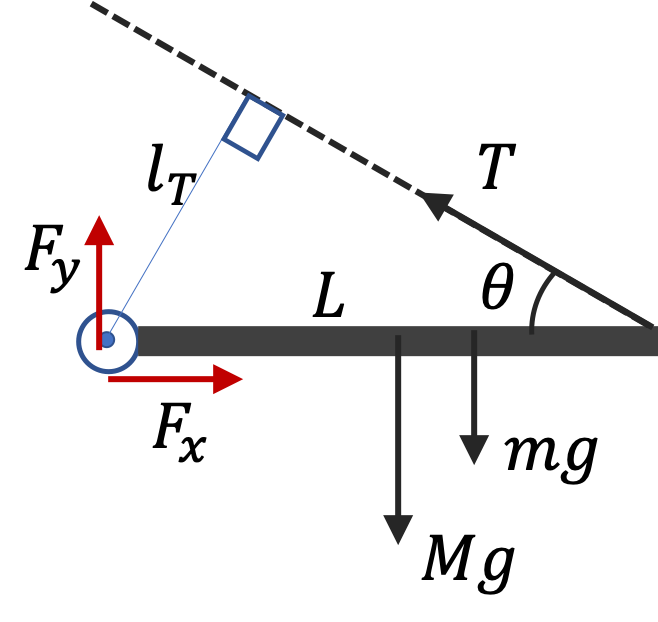Balancing the components of the forces we get
\begin{align} \amp F_x - T\cos\,\theta = 0, \label{eq-hanging-sign-post-Fx}\tag{16.1.30}\\ \amp F_y + T\sin\,\theta - mg - Mg = 0. \label{eq-hanging-sign-post-Fy}\tag{16.1.31} \end{align}
The weight of the beam can be taken to act the center, giving the lever arm $L/2\text{.}$ The sign is at a distance $d =2\text{ m}$ from the pin, giving it a lever arm of $d\text{.}$ The lever arm od tension about the pin is $l_T = L\sin\,\theta\text{.}$
\begin{equation} T\, l_T - M g \dfrac{L}{2} - m g d = 0. \label{eq-hanging-sign-post-torque}\tag{16.1.32} \end{equation}
We can get $T$ from this equation.
\begin{equation*} T = \dfrac{1}{l_T}\, \left( M g \dfrac{L}{2} + m g d\right). \end{equation*}
Now, that we have $T\text{,}$ we can use this in Eq. (16.1.30) to get $F_x\text{,}$
\begin{equation*} F_x = T\cos\,\theta, \end{equation*}
and $F_y$ from Eq. (16.1.31).
\begin{equation*} F_y = -T\sin\,\theta + mg + Mg. \end{equation*}
Now, we work out and/or list the numerical values.
\begin{align*} \amp L = 3\text{ m},\ \ d = = 2\text{ m},\ \ \theta = 30^\circ, \\ \amp l_T = L\sin\,\theta = 3 \times \sin\,30^\circ = 1.5\text{ m},\\ \amp Mg = 50\times 9.81 = 490.8\text{ N},\ \ mg = 300\times 9.81 = 2,943\text{ N}. \end{align*}
These will give
\begin{align*} \amp T = \dfrac{1}{1.5}\, \left( 490.8 \times 1.5 + 2,943 \times 2\right) = 4,415\text{ N}\\ \amp F_x = 4,415\times \cos\,30^\circ = 3,823\text{ N},\\ \amp F_y = - 4,415\times \sin\,30^\circ + 490.8 + 2,943 = 1,226\text{ N}. \end{align*}
Combining the components of $F\text{,}$ we get the magnitude of the force by the pin to be
\begin{equation*} F = \sqrt{F_x^2 + F_y^2} = 4,015\text{ N}. \end{equation*}
Solution 2 (b)

From $F_x = 3,823\text{ N}$ and $F_y = 1,226\text{ N}\text{,}$ we get the direction of the force by the pin to by the following counterclockwise angle with respect to the postive $x$ axis.

\begin{equation*} \theta = \tan^{-1} \left( \dfrac{1,226}{3,823} \right) = 17.8^\circ. \end{equation*}

A $20\text{-kg}$ wooden plank of length $2\text{ m}$ is resting against a frictionless support at an angle $30^{\circ}$ as shown. The distance from the free end to the support is $0.25\text{ m}\text{.}$ Find force(s) on the plank by the support and by the floor.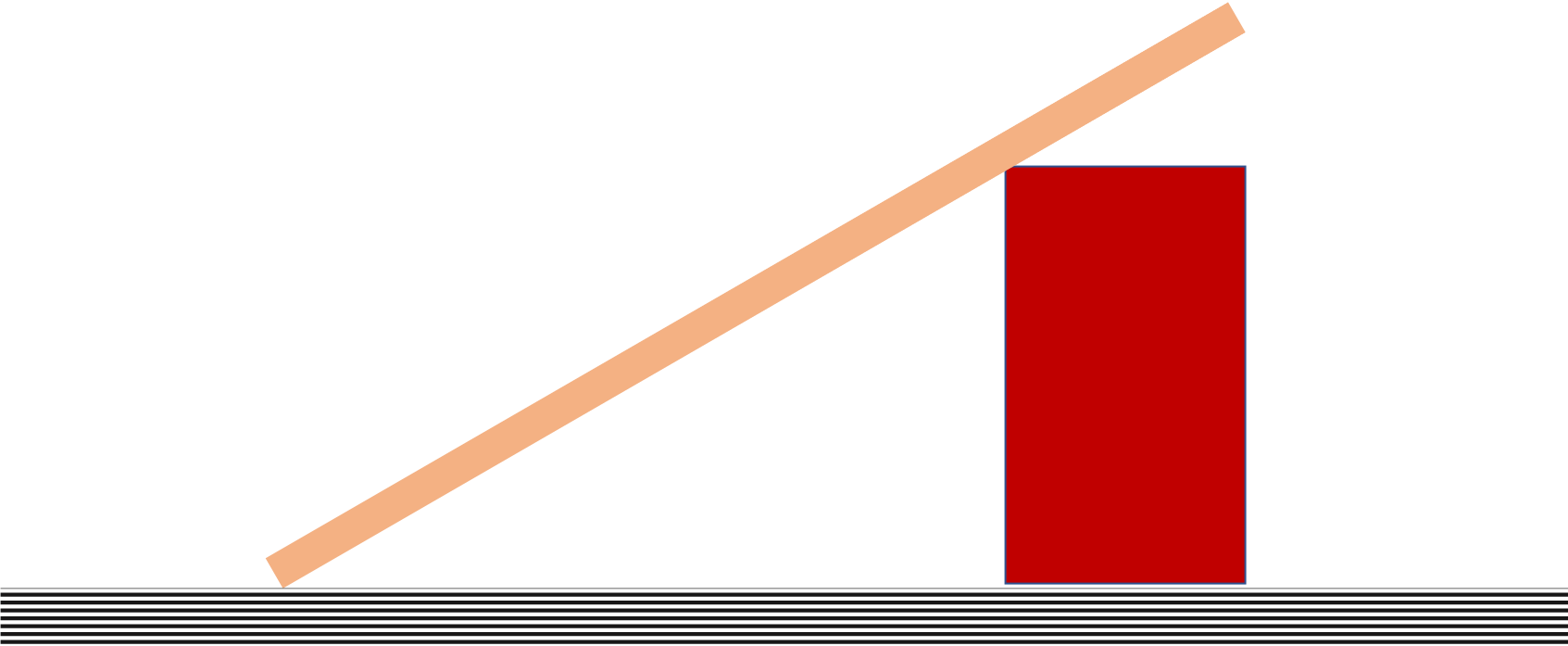Hint

1. Force from support is normal to the plank. (2) Balance torque about the point touching the floor first. (3) Then, use the balancing of forces along horizintal and vertical directions.

$S = 56.1\text{ N}\text{,}$ $F = 28.0\text{ N}\text{,}$ $N = 148.0\text{ N}\text{.}$

Solution

Let $S$ denote the force from the support, $N$ for normal from the floor, and $F$ the static friction from the floor. Force $S$ is perpendicular to the plank since there is no friction there and therefore, the support applies the normal force. Figure 16.1.14 summarizes the forces on the plank.

We will write the torques equation about an axis through the point at the corner labeled O. The lever arms are

\begin{align*} \amp r_{\perp,N} = r_{\perp,F} = 0, \\ \amp r_{\perp,W} = \dfrac{L}{2}\,\sin\,\theta,\\ \amp r_{\perp,S} = L-d. \end{align*}

All of these are obtained by drawing perpendiculars to the lines of forces. The torque balance equation gives

\begin{equation*} S (L-d) - Mg\dfrac{L}{2}\,\sin\,\theta = 0. \end{equation*}

Solving for $S$ we get

\begin{equation*} S = \dfrac{L}{2(L-d)} Mg\,\sin\,\theta. \end{equation*}

To get $N$ and $F\text{,}$ we balance forces along horizontal and vertical directions. For this we need to compute the componets of $S\text{.}$ Let positive $x$ be pointed right and positive $y$ pointed up.

\begin{equation*} S_x = - S\, \sin\,\theta, \ \ S_y = S\,\cos\,\theta. \end{equation*}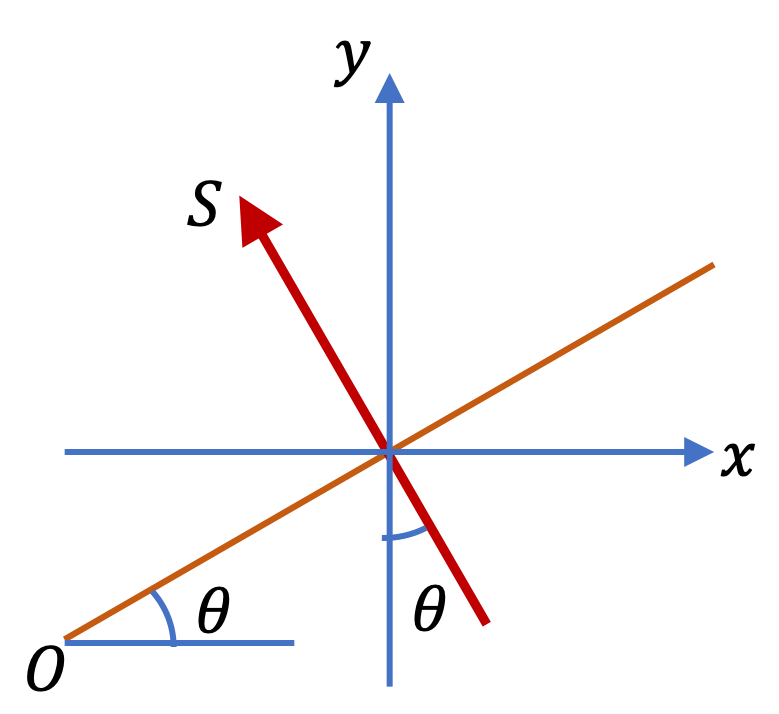The balancing of forces give us the following two equations.

\begin{align} \amp F + S_x = 0, \tag{16.1.33}\\ \amp N - W + S_y = 0\tag{16.1.34} \end{align}

Therefore, we have the following answer for the three forces required.

\begin{align*} S \amp= \dfrac{L}{2(L-d)} Mg\,\sin\,\theta. \\ F \amp = S\, \sin\,\theta, \\ N \amp = Mg - S\,\cos\,\theta. \end{align*}

Numerical values give

\begin{align*} S \amp= \dfrac{2}{2(2-0.25)} 20\times 9.81 \,\sin\,30^{\circ} = 56.1\text{ N}. \\ F \amp = 56.1/2 = 28.0\text{ N}, \\ N \amp = 20\times 9.81 - 56.1\,\cos\,30^\circ = 148\text{ N}. \end{align*}

A bar AB of mass $M$ and length $L$ is hinged at one end and supported by a rigid support at point C so that the bar hangs at angle $\theta$ as shown in the figure. The distance AC equals $f L\text{,}$ with $0\le f \le 1\text{.}$ Assume that the force from support C is perpendicular to the bar.

If you wish to work with numerical values: $M=100\text{ kg}\text{,}$ $F=600\text{ N}\text{,}$ $f=0.75\text{,}$ $\theta = 30^{\circ}\text{.}$ You do not need the value of $L\text{.}$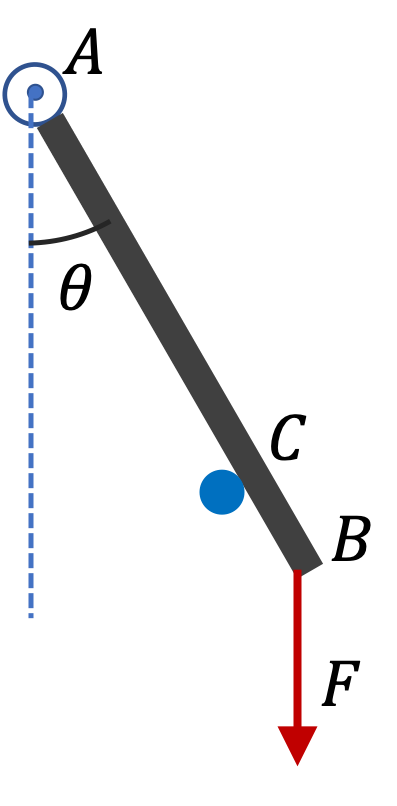Hint

$C = 727\text{ N}\text{,}$ $A_x = - 630\text{ N},\ \ A_y = 1,217\text{ N}.$

Solution

First we need to identify forces on the bar and balance torques and/or forces. We denote the forces at A and C as shown in the figure to the right.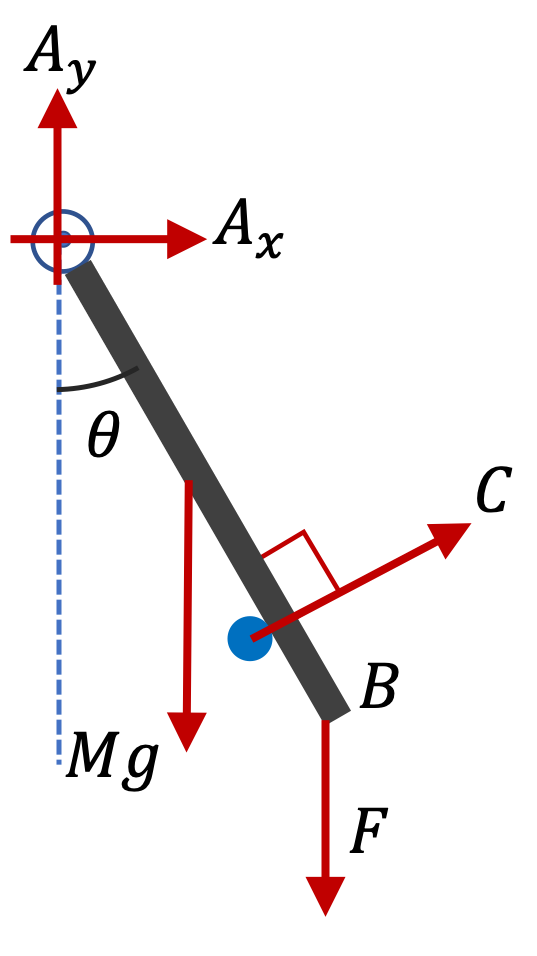Figure 16.1.16 help us compute the lever arms about the hinge at A.

Balancing the torque about A we get

\begin{equation*} C f L - M g \dfrac{L}{2}\,\sin\,\theta - F L\,\sin\,\theta = 0. \end{equation*}

This solves for $C\text{.}$

\begin{equation*} C = \dfrac{1}{2f}\, M g \,\sin\,\theta + \dfrac{1}{f}\, F \,\sin\,\theta. \end{equation*}

Now, balancing horizontal and vertical components of forces we get

\begin{align*} \amp A_x + C\,\cos\,\theta = 0. \\ \amp A_y + C\,\sin\,\theta - M g - F = 0. \end{align*}

These give

\begin{align*} \amp A_x = - C\,\cos\,\theta. \\ \amp A_y = - C\,\sin\,\theta + M g + F. \end{align*}

Numerical values give

\begin{align*} \amp C = 727\text{ N},\\ \amp A_x = - 630\text{ N},\ \ A_y = 1,217\text{ N}. \end{align*}

Figure 16.1.18 shows a rectangular box of cross-sectional dimensions $a\times b$ on an inclined surface whose angle of incline $\theta$ can be varied. If $\mu_s \gt \tan\theta\text{,}$ the box will not slide. Even when box would not slide, at some angle $\theta_c\text{,}$ box will tip over. Find this angle.

Hint

Torque about bottom right corner will not be balanced if angle is larget than the critical angle.

$\tan^{-1}\left( b/a\right)\text{.}$

Solution

Figure 16.1.19 shows force diagrams on the box when the incline is (a) at critical angle and (b) at an angle greater than the critical angle. At the critial angle, the weight vector is pointed towards the bottom right corner of the box. At higher angles, weight vector is to the right of the bottom right corner, which makes balancing of torque of the three forces impossible.

From the triangle in Figure 16.1.19(a), we see that

\begin{equation*} \tan\theta_c = \frac{b}{a}. \end{equation*}

Therefore,

\begin{equation*} \theta_c = \tan^{-1}\left( b/a\right). \end{equation*}

A football (spherical shape) of radius $R$ is kept on a hole of radius $r (r \lt R)$ made on a plank kept horizontally. One end of the plank is now lifted so that it gets tilted making an angle $\theta$ from the horizontal as shown in Figure 16.1.21. Find the maximum value of $\theta$ so that the football does not start rolling down the plank. (Adapted from Indian JEE, 2020.)

Hint

Torques from two normals and weight will not be balanced if weight of the ball passes to the right of the bottom corner.

$\sin^{-1}(r/R)\text{.}$

Solution

Figure 16.1.22 shows force diagram. The two normals are pointed towards the center of the ball and weight is pointed down. At the maximum angle before rolling would start, normal from the bottom point will be pointed vertical up as shown.

In the figure, I have introduced angle $\phi$ for convenience. This angle is related to incline $\theta$ by

\begin{equation*} \phi = 90^\circ - \theta. \end{equation*}

From the triangle with sides $R,\ R, 2r$ and angle $\phi\text{,}$ we immediately get that

\begin{equation*} R^2 + (2r)^2 - 2R(2r)\cos\phi = R^2. \end{equation*}

Therefore

\begin{equation*} \cos\phi = \frac{r}{R}. \end{equation*}

This is same as

\begin{equation*} \sin\theta = \frac{r}{R}. \end{equation*}

Therefore, the max angle is

\begin{equation*} \theta = \sin^{-1}(r/R). \end{equation*}

A cylindrical log of mass $M$ and radius $R$ is pushed over a step of height $a \lt R$ as shown in the Figure 16.1.24 with increasing amount of force $F\text{.}$ What is the value of the force at which the log will start to move over?

Hint

Normal from floor will be zero.

$\frac{\sqrt{R^2 - (R-a)^2}}{2R}\; Mg\text{.}$

Solution

Figure 16.1.25(a) shows the situation before the final value of $F\text{.}$ As you increase $F\text{,}$ the normal from floor decreases and at the critical value of $F\text{,}$ as shown in (b), the normal from floor vanishes.

Figure 16.1.25(b) shows the lever arms of $Mg$ and $F$ from the tip of the step. The lever arms will be

\begin{equation*} r_{\perp w} = \sqrt{R^2 - (R-a)^2},\ \ \ r_{\perp F} = 2R. \end{equation*}

Balancing torque in the situation in Figure 16.1.25(b) gives

\begin{equation*} F r_{\perp F} = Mg r_{\perp w}, \end{equation*}

which gives

\begin{equation*} F = \frac{\sqrt{R^2 - (R-a)^2}}{2R}\; Mg. \end{equation*}

In Checkpoint 16.1.23, find the magnitude and direction of the push on the log from the tip of the step at the instant the log begins to move over.

Hint

Balance the forces on the log.

Solution

No solution provided.